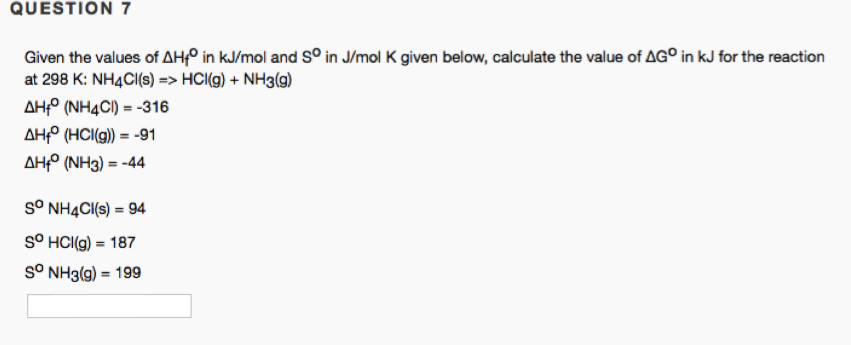# Given the values of AHf° in kJ/mol and S° in Jimol K given below, calculate the value of AG° in kJ for the reaction at 298 K: NH4C1(s) => HCI(g) + NH3(g) AHf° (NH4CI) = -316 AHf° (HCI(g)) = -91 AHf° (NH3) = -44 S° NH4CI(s) = 94 S° HCI(g) = 187 S° NH3(g) = 199